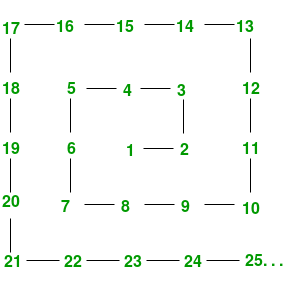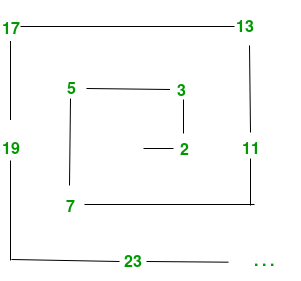# The Ulam Spiral

• Difficulty Level : Hard
• Last Updated : 08 Feb, 2018

The Ulam spiral or prime spiral is a graphical depiction of the set of prime numbers. It emphasizes the striking appearance in the spiral of prominent diagonal, horizontal, and vertical lines containing large numbers of primes.

How to construct Ulam Spiral?
The number spiral is constructed by writing the positive integers in a spiral arrangement on a square lattice, as shown.

Attention reader! Don’t stop learning now. Get hold of all the important mathematical concepts for competitive programming with the Essential Maths for CP Course at a student-friendly price. To complete your preparation from learning a language to DS Algo and many more,  please refer Complete Interview Preparation Course.The Ulam spiral is produced by specially marking the prime numbers—for example by circling the primes or writing only the primes or by writing the prime numbers and non-prime numbers in different colors—to obtain a figure like the one below.```Code to Generate Prime Number
#importing the required libraries
import math
import itertools

def primes():
yield 2
primesSoFar = 
for candidate in itertools.count(3, 2):
for prime in (i for i in primesSoFar if i  100:
break
print(p)

if __name__ == "__main__":
main()

```

But that’s not what we wanted. We wanted to generate Ulam spiral, isn’t it?
Its just getting started so the next step is to generate the prime spiral ; to generate one pixel for each natural number, black or white, depending on whether the number is a prime or not. To generate such plot we will use the matplotlib library of python and numpy for mathematical purposes.

So now the question is how should we approach? It’s quite a simple idea. First we have to generate a array of prime numbers of size ‘n^2’. Then create an array of boolean values: 1 for prime, 0 for composite of same size ‘n^2’. The next step should be convert the second array of boolean values to spiral the values clockwise out from the center and to do so we can proceed with the following steps :

1: Generate an array with elements containing ‘n*n’ and then reshape the array to matrix form of order ‘n X n’.

2: Create another empty list then append the first row of the matrix generated in step 1.

3: Remove the first row (the one we’ve just appended to spiral).

4: Rotate the rest of the array anticlockwise.

5: Make a flat array of indices spiralling into the array.

6: Index into a flattened version of our target array with spiral indices.

And after following the steps you are good to go and lastly just plot the array we got after the following operations using matplotlib as mentioned above. The code is given down below still i would highly recommend you to try this out first by yourself then dig in to the code.

## Recommended: Please try your approach on {IDE} first, before moving on to the solution.

 `# Python code to print Ulam's spiral``import` `numpy as np``import` `matplotlib.pyplot as plt``import` `matplotlib.cm as cm`` ` `# function to plot out the ulam spiral``def` `make_spiral(arr):``    ``nrows, ncols``=` `arr.shape``    ``idx ``=` `np.arange(nrows``*``ncols).reshape(nrows,ncols)[::``-``1``]``    ``spiral_idx ``=` `[]``    ``while` `idx.size:``        ``spiral_idx.append(idx[``0``])`` ` `        ``# Remove the first row (the one we've``        ``# just appended to spiral).``        ``idx ``=` `idx[``1``:]`` ` `        ``# Rotate the rest of the array anticlockwise``        ``idx ``=` `idx.T[::``-``1``]`` ` `    ``# Make a flat array of indices spiralling ``    ``# into the array.``    ``spiral_idx ``=` `np.hstack(spiral_idx)`` ` `    ``# Index into a flattened version of our ``    ``# target array with spiral indices.``    ``spiral ``=` `np.empty_like(arr)``    ``spiral.flat[spiral_idx] ``=` `arr.flat[::``-``1``]``    ``return` `spiral`` ` `# edge size of the square array.``w ``=` `251``# Prime numbers up to and including w**2.``primes ``=` `np.array([n ``for` `n ``in` `range``(``2``,w``*``*``2``+``1``) ``if` `all``(``                        ``(n ``%` `m) !``=` `0` `for` `m ``in` `range``(``2``,``int``(np.sqrt(n))``+``1``))])`` ` `# Create an array of boolean values: 1 for prime, 0 for composite``arr ``=` `np.zeros(w``*``*``2``, dtype``=``'u1'``)``arr[primes``-``1``] ``=` `1`` ` `# Spiral the values clockwise out from the centre``arr ``=` `make_spiral(arr.reshape((w,w)))`` ` `plt.matshow(arr, cmap``=``cm.binary)``plt.axis(``'off'``)``plt.show()`

For a better understanding of this mathematical concept prefer refer to this video.

This article is contributed by Subhajit Saha. If you like GeeksforGeeks and would like to contribute, you can also write an article using contribute.geeksforgeeks.org or mail your article to contribute@geeksforgeeks.org. See your article appearing on the GeeksforGeeks main page and help other Geeks.